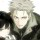# WordPress连接微博专业版（一键登录网站，同步到微博、博客，社会化评论）

## 简要描述

WordPress连接微博 是由“水脉烟香”开发的第一个Wordpress插件。插件于2011年1月20日发布第1版，包括社交帐号一键注册登录网站，同步文章到微博、博客，本地化的社会化评论系统等功能。通过这个插件，您可以更简单的推广您的网站、产品，最大化曝光在社会化媒体阵营。它不经过任何第三方服务器，更加安全、稳定、高效。

## 加入我们

QQ群讨论：88735031149451879（验证信息：wp）

QQ咨询: 3249892

## 专业版功能

1、使用QQ、新浪微博、微信等16个社交帐号登录您的网站。

2、同步文章到微博/SNS，可以同步第一张图片和视频。

3、支持自定义APP key
4、本地化“社会化评论框”，包括微博评论回推到网站和新回复邮件通知。（当前回推功能不稳定，介意者不要购买！）
5、社交数据统计（包括注册/登录/同步评论等），社交用户分析（认证用户|社交网站|性别|地区分布等）
6、同步全文或者部分内容到其他博客。

7、使用社会化分享按钮。
8、写文章/发微博WAP页面（适用于手机浏览器）
9、隐藏文章的部分或者全部内容，用户通过登录、回复、分享等行为后才能显示隐藏的内容。
10、支持wp后台自动升级。
11、完美支持https网站。

## 点击查看安装及使用文档

WordPress连接微博捐赠版用户(2013年1月3日前捐赠的用户)购买WordPress连接微博专业版插件享受5折优惠。输入捐赠时填写的网址即可看到优惠。## 插件问题

1、对服务器有什么要求?

PHP版本不限制（支持PHP 5.2~PHP 8），但是服务器需要安装curl组件才能使用社交登录和同步功能。

2、启用插件时出现如下错误： ..xxx.php:3

3、我想修改微博信息下面的来自xxx，怎么做？

4、不能同步到新浪微博，解决方法：

(1). 如果以前同步过，可能是微博授权过期了，重新绑定微博即可。
(2). 如果你正在使用西部数据的主机，请联系他们关闭CDN即可。
(3). 可能是您的网站图片出问题了，您可以发布纯文字试试，如果纯文字能同步，那就是图片有问题，请检查是否设置了黑名单或者防火墙。请把以下几个网址加入到允许访问的范围内：

```https://api.weibo.com
http://open.t.qq.com
http://api.t.163.com
http://api.t.sohu.com
https://graph.qq.com
http://api.renren.com
https://api.kaixin001.com
http://api.renjian.com
http://wbto.cn
```

5、是否支持定时的文章同步

6、想要同步到QQ空间日志，要做什么准备？

7、是否支持使用离线发布工具的文章同步

8、是否支持采集的文章同步?

## 售后问题

1、是一次性收费还是每年收费？我能免费升级吗?

2、我能退货吗?

3、我用了一段时间后，能更换域名吗？

4、提供技术支持吗?

5、我应该选择单域名/根域名/WPMU中的哪一类型?

6、安装时应该注意什么？

## 插件历程

1、2011/1/20 发布第1版，当时的名称叫“Wordpress微博同步”。
2、2011/2/22 修改插件名称为 “WordPress连接微博”，增加了登录功能。
3、2011/4/26 发布捐赠版本。（V1.4）
4、2011/11/14 与灯鹭网合作，集成灯鹭接口，V2.x 版权归灯鹭网。
5、2013/1/3 感谢400多位热心用户的捐赠和支持，Wordpress连接微博 及高级设置版插件变得越来越强大。遗憾的是，插件开发者投入了大量时间、精力和金钱，无奈之下决定收费，愿广大新老客户予以理解，并能一如既往地支持。再次衷心感谢！
6、2013/1/28 发布付费插件“Wordpress连接微博 专业版”（V3.0），由Wordpress连接微博 高级设置版演变而来。

ps:免费版由于长期未更新，而且灯鹭团队已解散，已经无法正常使用。

## 更新日志

```= 4.7.2 =

2022/7/17

= 4.7.1 =

2021/7/15

= 4.7 =

2020/12/29

= 4.6.9 =

2019/8/14

= 4.6.8 =

2019/6/22

= 4.6.7 =

2018/12/16

= 4.6.6 =

2018/9/15

= 4.6.5 =

2018/8/8

= 4.6.4 =

2018/6/28

= 4.6.3 =

2018/4/12

= 4.6.2 =

2017/8/15

= 4.6.1 =

2017/7/5

= 4.5.1 =

2017/3/6

= 4.5 =

2017/3/5

= 4.4.1 =

2016/9/27

= 4.4 =

2016/8/18

= 4.3 =

2016/6/29

= 4.2.4 =

2016/4/18

= 4.2.3 =

2016/3/11

= 4.2.2 =

2016/2/20

= 4.2.1 =

2015/12/20

= 4.2 =

2015/12/14

= 4.1.5 =

2015/9/23

= 4.1.4 =

2015/8/2

= 4.1.3 =

2015/7/10

= 4.1.1 =

2015/7/9

= 4.1 =

2015/7/3

= 4.0.3 =

2015/5/14

= 4.0.2 =

2015/5/13

= 4.0.1 =

2015/5/10

= 4.0 =

2015/5/8

= 3.6.11 =

2015/03/23

= 3.6.10 =

2015/03/20

= 3.6.9 =

2015/01/29

= 3.6.8 =

2014/11/22

= 3.6.7 =

2014/11/20

= 3.6.6 =

2014/11/02

= 3.6.5 =

2014/09/26

= 3.6.3 =

2014/09/17

= 3.6.2 =

2014/07/24

= 3.6.1 =

2014/07/11

= 3.6 =

2014/07/07

= 3.5.8 =

2014/05/28

= 3.5.7 =

2014/05/27

= 3.5.6 =

2014/05/24

= 3.5.5 =

2014/05/09

= 3.5.3 =

2014/05/03

= 3.5.2 =

2014/04/08

= 3.5 =

2014/04/05

= 3.4.3 =

2014/02/20

= 3.4 =

2014/01/20

1、使用社交帐号登录并发布评论，可以选择是否同步到微博。
2、微博评论回推到网站。
3、管理员或者文章作者可以置顶评论，让重要评论不再沉下去。
4、顶踩评论，点击“最热”排序，不错过优质评论。
5、作者的评论和回复会标注为“文章作者”,妈妈再也不担心被恶意评论骗了。
6、提供两套模板，会陆续加入更多评论模板，也欢迎大家贡献模板。

= 3.3 =

2013/10/12

= 3.2.9 =

2013/9/23

= 3.2.8 =

2013/9/18

= 3.2.6 =

2013/8/3

= 3.2.5 =

2013/6/3

= 3.2.4 =

2013/5/22

= 3.2.3 =

2013/4/20

= 3.2.2 =

2013/4/12

= 3.2.1 =

2013/3/25

QQ登录用户名调整

= 3.2 =

2013/3/22

= 3.1 =

2013/2/25

= 3.0 =

2013/1/28

```

## 插件早期捐赠名单1. 评论框CSS样式修改：http://www.smyx.net/pinglun-css.html

史上最全的Wordpress连接微博专业版文档：https://wptao.com/doc/wp-connect.html

2014年1月7日 23:59
2. 请问这个插件支持从自定义字段中读取内容同步到微博吗？

2014年1月4日 21:11来自新浪微博
• 同问

2014年5月3日 23:35来自新浪微博
• 回复@电商疯人院:。。。

2014年5月3日 23:36来自新浪微博
• 可以，但是要写几句代码实现这个功能，把下面代码复制到主题的functions.php内，代码如下：

```// 从自定义字段获取文本内容 function sync_weibo_for_post_meta(\$list, \$post_ID, \$post_author_ID) { \$box = '改成自定义字段名称'; \$text = \$_POST[\$box] ? \$_POST[\$box] : get_post_meta(\$post_ID, \$box, true); if (\$text) { \$list['content'] = \$text; \$list['text'] = '【' . \$list['title'] . '】' . \$text; } return \$list; } add_filter('post_sync_weibo', 'sync_weibo_for_post_meta', 10, 3);```

2014年1月5日 13:52
• 加了这段代码可以同步自定义段，不过文章内容没有同步，能不能同时同步文章内容然后附带上自定义字段？

2014年3月28日 21:55来自新浪微博
• 回复@水脉烟香:如果读取图片可以吗，读取自定义字段中图片的url，作为微博的配图？

2014年1月5日 18:47来自新浪微博
• 当然可以，插件拥有良好的扩展功能，依然要写几句代码实现这个功能，把下面代码复制到主题的functions.php内，代码如下：

```// 从自定义字段获取图片 function get_thumb_for_post_meta(\$none, \$content, \$post_ID) { \$box = '改成自定义字段名称'; \$image = \$_POST[\$box] ? \$_POST[\$box] : get_post_meta(\$post_ID, \$box, true); if (\$image) { return array(\$image); } } add_action('wp_multi_media_url', 'get_thumb_for_post_meta', 10, 3);```

2014年1月6日 14:04
3.超嘎

评论可以用超链接吗？ https://fouzhai.com

5月9日 00:32
4.kanpk123

分享到微博，不显示特色图片，而且链接也只有一半怎么办

2月9日 09:16
5.codihuang

请问这个插件，目前还能用吗？

1月9日 12:42
•超嘎

还可以用

5月9日 00:29
6.六丈江湖

文章中若有图片，则同步不成功，并显示如下报错：
“新浪微博错误提示：
帐号：六丈江湖
描述：10008 : picid is null !
API：/2/statuses/share.json”

经排查，关掉CDN的Referer防盗链功能，就可以同步图片，不会报错。但因自己要使用防盗链，请问我应该将什么地址加入白名单？

2022年8月1日 22:31